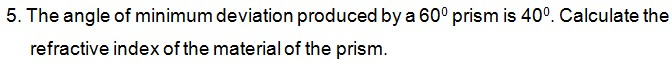#9 years ago

Here refractive angle(A)=60 , angle of minimum deviation(B)=40 and find the refractive index(C)=?

so,

refractive index(C)=sin{(A+B)/2} / {sin(A/2)}

=sin{(60+40)/2} / {sin(60/2)}

=(sin50) / (sin30)

=(0.7660) / (1/2)

=(0.7660)(2) / (1)

=1.532